Question

# Problem 8. (1 point) (1 pt) Calculate all four second-order partial derivatives of f(x,y) = 2x²y...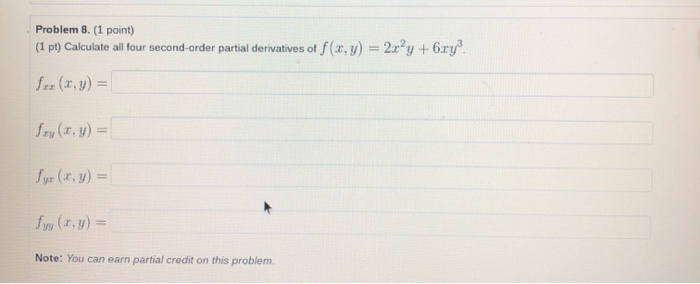Problem 8. (1 point) (1 pt) Calculate all four second-order partial derivatives of f(x,y) = 2x²y +6.ry? for (x,y) = Szy(x, y) = Syr (x, y) = fry (x. y) = Note: You can earn partial credit on this problem.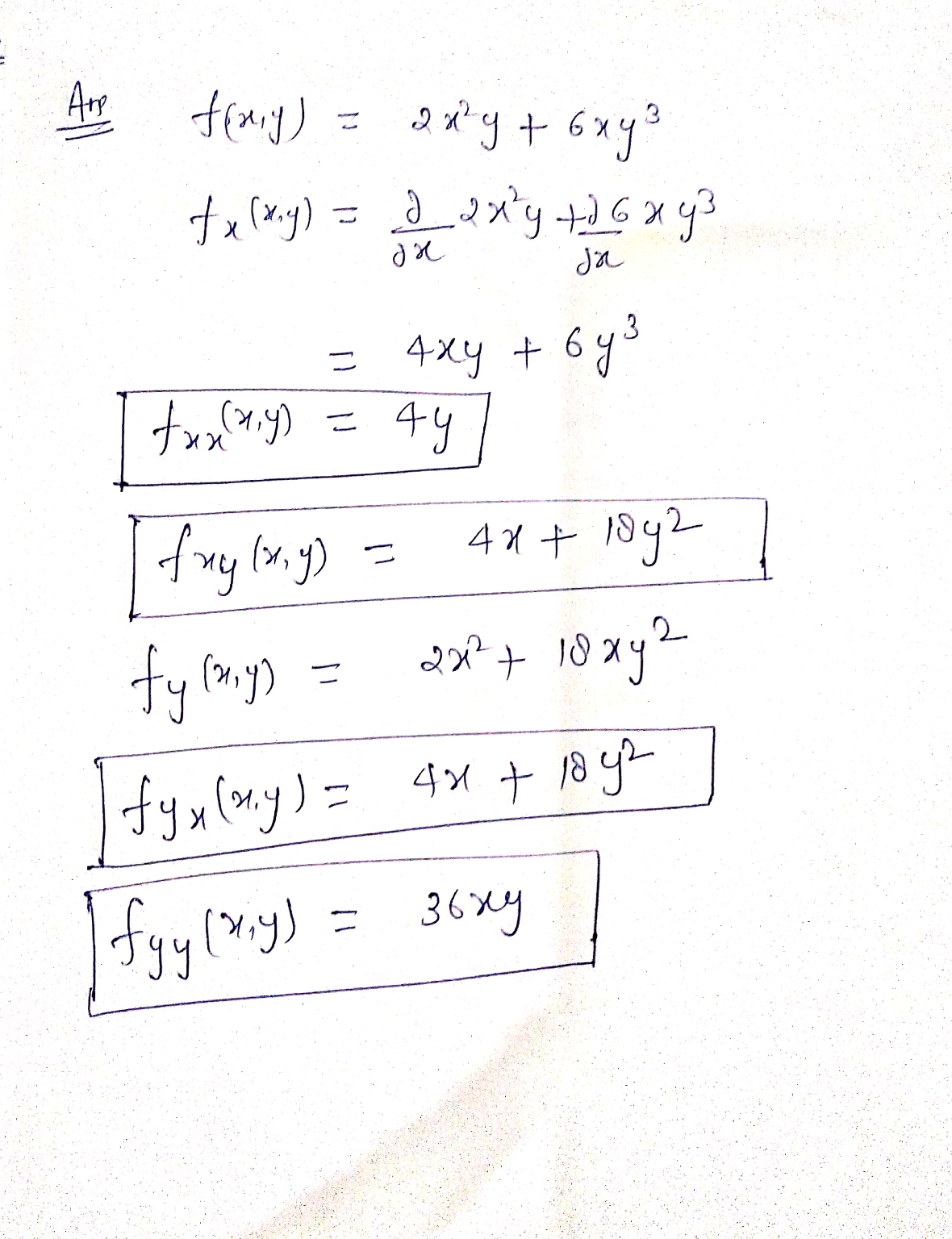#### Earn Coins

Coins can be redeemed for fabulous gifts.

Similar Homework Help Questions
• ### Problem 5. (1 point) Find all the first and second order partial derivatives of f(x,y) 7...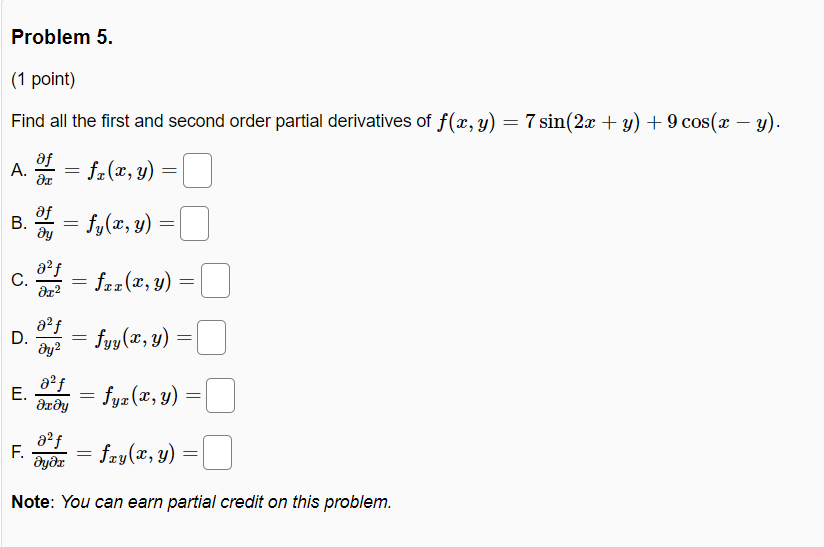Problem 5. (1 point) Find all the first and second order partial derivatives of f(x,y) 7 sin(2x + y) + 9 cos(x - y). A. = fx(x,y) = B. = fy(x, y) = af C. ar2 = fcz(x, y) = af D. ay2 = fyy(x,y) = E. af деду fyz(x, y) = af F. მყმz = fxy(x, y) = Note: You can earn partial credit on this problem.

• ### Find all the first and second order. partial derivatives of f(x, y) = 8 sin(2x +...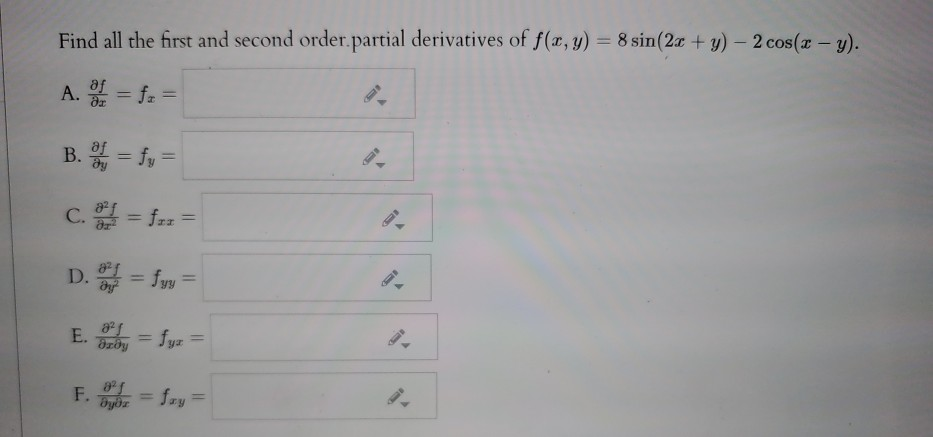Find all the first and second order. partial derivatives of f(x, y) = 8 sin(2x + y) - 2 cos(x - y). A. SI = fr = B. = fy = c. = f-z = D. = fyy = E. By = fyz = F. = Sxy=

• ### Calculate all four second-order partial derivatives and check that fty = fur. Assume the variables are...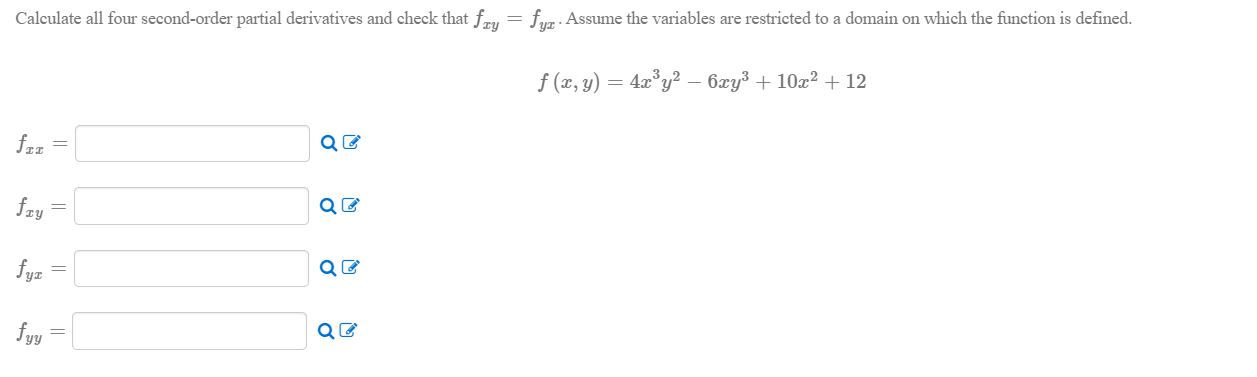Calculate all four second-order partial derivatives and check that fty = fur. Assume the variables are restricted to a domain on which the function is defined. f (x,y) = 4.xºy2 – 6xy3 + 10x2 + 12

• ### Find all second order partial derivatives of f(x, y) = (x² +3y)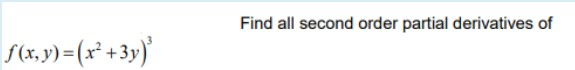Find all second order partial derivatives of f(x, y) = (x² +3y)

• ### (10 points) Find all first and second partial derivatives of f(x,y) = 24 – 3.z”y2 +...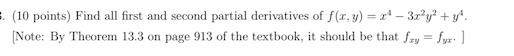(10 points) Find all first and second partial derivatives of f(x,y) = 24 – 3.z”y2 + y* [Note: By Theorem 13.3 on page 913 of the textbook, it should be that fry = fyr:]

• ### Find the four second-order partial derivatives for f(x, y) = 2x4,5 + 7x®y?. tyy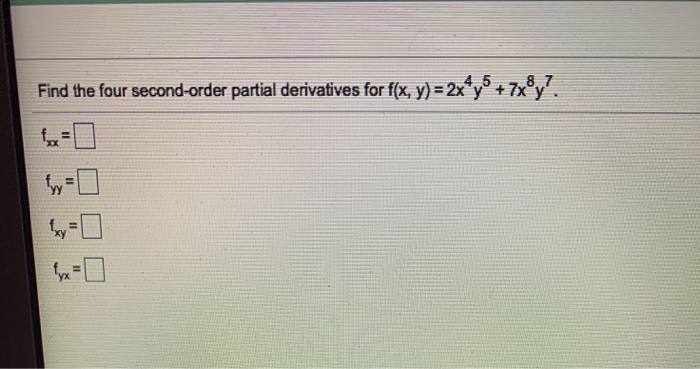Find the four second-order partial derivatives for f(x, y) = 2x4,5 + 7x®y?. tyy

• ### For questions 3-8: 5y2 Let f(x, y) = + y 2 Find the two first partial...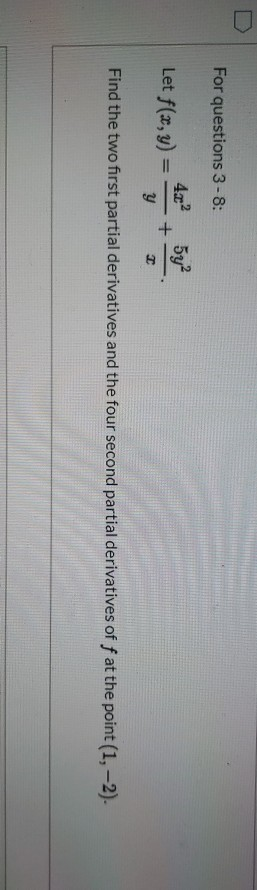For questions 3-8: 5y2 Let f(x, y) = + y 2 Find the two first partial derivatives and the four second partial derivatives of f at the point (1, -2). Question 6 Find fry (1,-2). Question 7 Find fy (1, -2). D Question 8 Find fy: (1, -2).

• ### 3. Find all second partial derivatives of f(x,y) = ry - 3ry2 + 2y2,2 4. Find...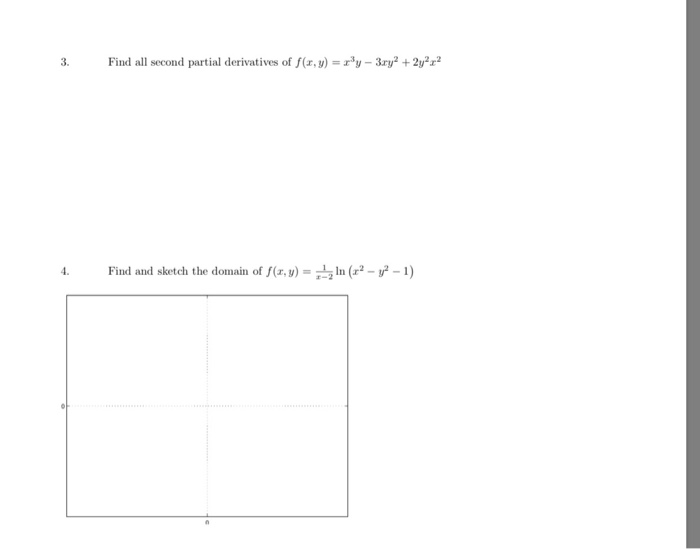3. Find all second partial derivatives of f(x,y) = ry - 3ry2 + 2y2,2 4. Find and sketch the domain of f(x,y) = . In (?-?-1)

• ### (1 point) Consider the function defined by F(x, y) = x2 + y2 except at (r, y) - (0, 0) where F(0,0)0 Then we have (0,0)...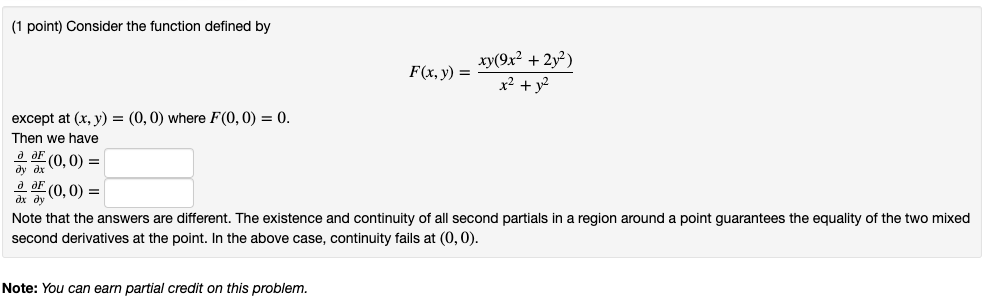(1 point) Consider the function defined by F(x, y) = x2 + y2 except at (r, y) - (0, 0) where F(0,0)0 Then we have (0,0) = (0,0) = ax dy Note that the answers are different. The existence and continuity of all second partials in a region around a point guarantees the equality of the two mixed second derivatives at the point. In the above case, continuity fails at (0,0) Note: You can earn partial credit on this problem...

• ### Let f(x,y) = (x" + 2?y?)!. compute all second-order partial derivatives of fat (0,0), if they...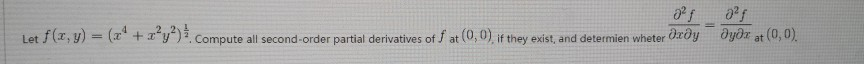Let f(x,y) = (x" + 2?y?)!. compute all second-order partial derivatives of fat (0,0), if they exist, and determien wheter dæðyəyər at (0,0).